Historical Archive of the Bible Wheel Site

The Bible Wheel had been debunked by its author.
Read all about it: Debunking Myself: What A Long Strange Trip It's Been

Recent Blog Articles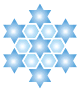For we are labourers together with God: ye are God’s husbandry, ye are God’s building. According to the grace of God which is given unto me, as a wise masterbuilder, I have laid the foundation, and another buildeth thereon. But let every man take heed how he buildeth thereupon. For other foundation can no man lay than that is laid, which is Jesus Christ.

I Corinthians 3.9f

The Holographic Generating set consists of three geometrically integrated Numbers: the Third Cube, 27, and the Fourth Hexagon/Star pair, 37/73.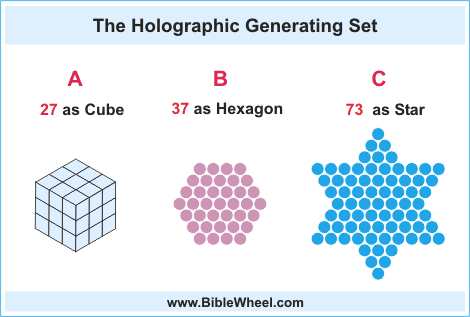The central Number 37 is geometrically related to both 27 and 73. It relates to 73-as-Star by representing its hexagonal core, and to 27-as-Cube by being the difference of consecutive cubes:

37 = 64 - 27 = 43 - 33

Alternately, the Number 27 can be seen as the sum of the first three centered Hexagonal numbers, Hex(1) + Hex(2) + Hex(3) = 1 + 7 + 19 = 27 (see Cubic Projections). The interplay of these three geometric numbers, and their integration with Genesis 1.1 is discussed at length in many insightful articles found on Vernon Jenkins' site, such as Squaring The Cubes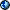and The Lamp.

The Number 37 may also be understood as the diagonal projection of a Cube constructed of 64 Unit Cubes. It is also bifigurate, meaning that it can be represented as either the third Star or the Fourth Hexagon. Such numbers are very rare. The next on after 37 is 1261, and there are only 12 such numbers less than 200 trillion (they are listed in 37's article. To find this rare, beautiful, multifaceted prime in the very heart of the holographic structure of Holy Scripture is perhaps the surest sign of the God's direct involvement of its design.

To behold the unmitigated power of the Generating Set, we need only look at the first verse of the Bible, the Genesis 1.1 component of the Creation HyperHolograph:

Genesis 1.1 = 2701 = 37 x 73 = Sum(73) = Sum(37) + 2 x 27 x 37

This equatation represents the profound triangular structure and self-reflective triangular substructure of the Genesis 1.1 Holograph. Except for the factor of 2, it is written entirely in terms of the GenSet. Unfortunately, it can be hard to see through the plethora of algebraic signs and numbers; this one line contains a total of 44 symbols. To reduce the clutter, I label the three elements:

A = 27    B = 37    C = 73

This will take a little strain off the eyes, and allow us to follow the symbolic flow of the GenSet through the holographs. It is easy enough to plug the values back in whenever necessary. To further simplify the expressions, we will use the standard mathematical practice of representing multiplication by simply juxtaposing the symbols, i.e.:

B x C = BC

This is the beauty of abstract symbols; this notation doesn't work with numbers since 3773 could be 3 x 773 or 37 x 73 or 377 x 3, or just 3773, while BC = 37 x 73 is perfectly unambiguous. Using these conventions, the lucidity of the Genesis 1.1 equation greatly increases:

Genesis 1.1 = 2701 = BC = Sum( C ) = Sum( B ) + 2AB

An immediate fact now leaps out - 2701 is simply 100A + 1, so we may write:

Genesis 1.1 = BC = 100A + 1

But 100 = A + C, so we can write:

Genesis 1.1 = BC = (A + C)A + 1 = A2 + AC + 1

We have now four simple ways to express Genesis 1.1 in terms of the GenSet. Note that these different formulas reflect different structural.phpects of the verse, as noted in the table:

 Generating Set: Genesis 1.1 Gen 1.1 = BC Prime composition of 2701. = Sum(C) Triangular Structure = Sum(B)+ 2AB Triangular Substructure = A2 + AC + 1 Self-reflection of the GenSet

The last identity is deeply integrated with the self-reflectivity of the GenSet as can be seen by contemplating some of the binary products from its elements (note the table includes a new symbol D = 137 = A + B + C, the sum of the elements):

 Selected Binary Products from Generating Set BC 37 x 73 2701 2700 + 1 A x 102 + 1 AD 27 x 137 3699 3700 - 1 B x 102 - 1 AA 27 x 27 729 730 - 1 C x 101 - 1 BB 37 x 37 1369 1370  - 1 D x 101 - 1 CD 73 x 137 10001 10000 + 1 ....104 + 1 AB 27 x 37 999 1000  - 1 ....103  - 1

The GenSet self-reflectivity mirrors the defining property of Biblical Holographs. It is also integrated with Base Ten, with many binary products differing by +/- 1 from multiples of Ten times elements of the GenSet. This is due in part to the termination of the elements with a 3 or 7, since this will always result in a product that ends in either a 1 or 9. There are also many other relations that arise from two powers of Ten being the sum and difference of GenSet elements:

A + C = 100 and B - A = 10

The reciprocals also reveal a deep self-reflective integration of the GenSet elements:

 Reciprocals of Generating Set Elements 1/A = 1/27 = 0.037037037037 ... repeating digits of B 1/B = 1/37 = 0.027027027027 ... repeating digits of A 1/C = 1/73 = 0.01369863013698630 ... repeating digits of B2 1/D = 1/137 = 0.007299270072992700 ... repeating digits of A2 and A

The GenSet is deeply integrated with fundamental concepts like Truth, Foundation, and Jesus Christ:

 Fundamentals A + B 64 Truth B - A 10 Base Ten A + C 100 Ten Squared B + C 110 Foundation A + B + C 137 The God of Truth AB 999 Ten Cubed - 1 AB + B2 2368 JESUS CHRIST

The last identity is particulary significant in light of the plain text of Scripture which declares Jesus Christ to be the only foundation. This is the foundation upon which we can build with great security: Jesus Christ, the eternal foundation, the Creator of All. The mysterious depths of His Name knows no end. Plugging the numbers back in, we have:

JESUS CHRIST = 2368 = 37 x 64

As mentioned above, the Numbers 37 and 64 are geometrically related - 37 is the diagonal projection of 64 - the Cube of Truth. It counts the number of visible Unit Cubes that combine to form the Fourth Cube, the Number 64. This means the the numerical weight of the name Jesus Christ may be understood as:

JESUS CHRIST = 37 (Visible Facet of Cube of Truth) x 64 (Cube of Truth)

This means that Jesus Christ is the product of the shadow and the substance the Cube of Truth. Glory!

The GenSet provides trmendous insight into the mathematical integration of Genesis 1.1 and John 1.1. We begin by recalling their similiar mathematical strutures (cf. Creation HyperHolograph: John 1.1). Both can be written as the product of a pair of palindromic factors:

 John 1.1 = 3627 = 39 x 93 Genesis 1.1 = 2701 = 37 x 73

The Numbers 3627 and 2701 are also linked by the the Number 3700, encountered above in the table of Binary Products (3700 = 100B = AD + 1). The simplicity of the connection is stunning:

 Genesis 1.1 + AB = 3700 = John 1.1 + C

This is an extremely simple relationship! The GenSet is distributed between Genesis (A, B) and John (C). Solving for John 1.1 in terms of Genesis 1.1 yields a simple expression of John 1.1 in terms of the GenSet:

John 1.1 = Genesis 1.1 + AB - C = BC + AB - C = 100B - C

This means we can represent the sum of Genesis 1.1 and John 1.1 in terms of the GenSet:

Genesis 1.1 + John 1.1 = 2BC + AB - C

Rearranging the factors (noting 2B - 1 = C) yields extremely simple expressions for both the sum and the difference of these two central verses which are nearly identical in form:

 John 1.1 -  Genesis 1.1 = AB - C John 1.1 + Genesis 1.1 = AB + C2

Now that we have arrived at the algebraic result, let us plug the numbers back in to see what we really have:

Genesis 1.1 + John 1.1 = 2701 + 3627 = 6328 = 732 + 999

There just one more fact to note - the Number 6328 is Triangular! Glory to God in the Highest! We have the identity:

Genesis 1.1 + John 1.1 = C2 + AB = Sum(112)

And what is the meaning of the Number 112? This is one of the most significant numbers in the Universe. It is the value of the fundamental Divine Name used throughout the Old Testament:

 The LORD God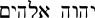YHVH Elohim = 112

This is built from the Names YHVH and ELOHIM that are integrated with the New Testament title The Father in the Unity Holograph! The Divine Name is generated from binary combinations of the Unity Holograph Generating Set (2, 13, 43):

YHVH = 26 = 2 x 13      ELOHIM = 86 = 2 x 43

Yet there is more! The Unity Holgraph reveals the fundamental significance of the Number 39 as:

 The LORD is One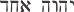YHVH Echad = 39 = 3 x 13 = 3 x ONE

The Number 39 combines with C = 73 to form the index of the Triangular sum of Genesis 1.1 and John 1.1:

 The LORD GodYHVH Elohim = 112 = C + 39

Putting this all together reveals the God's Holy Work in the supernatural design of Scriture and the Greek and Hebrew languages:

 Genesis 1.1 + John 1.1 = AB + C2 = Sum(THE LORD GOD)

Thus we can see all creation descending from the Name of THE LORD GOD!Yet for all this, we have just begun! The GenSet relation between Genesis 1.1 and John 1.1 also leads directly to a simple expression for John 1.1:

John 1.1 = Genesis 1.1 + AB - C

Substituting Genesis 1.1 = BC yields this elegent relation:

John 1.1 = AB + BC - C

Likewise, we can write John 1.1 in terms of D = A + B + C = 137. Recall from the table of Binary Products 3700 = 27 x 137 + 1 = AD + 1

John 1.1 = AD - C + 1

Now this is simplicity! Yet it gets even better! The sum of the entire Divine Prologue integrates with its central claim through the Number 137 = D (The God of Truth):

 All things weremade by him = 2055 = 15D John 1.1-5 = 26715 = 15D x 13

The GenSet also yields insight into the relation between John 1.1 and the Word (443) - their expressions are of very similar form:

 John 1.1 = 3627 = 3700  - 73 = 100B -  C The Word =  443 =   370 + 73 =  10B + C

The similar forms of John 1.1 and The Word immediately yields a simple expression of their sum:

John 1.1 (3627) + The Word (443) = 100B - C + 10B + C = 110B

Recalling 110 (Foundation) = B + C then yields this extremely simple relation between John 1.1, the Word, and Genesis 1.1:

John 1.1 + The Word = 110B = Genesis 1.1 + B2

Of course, B2 = 37 x 37 = 1369 = Image of God, which is one of the Titles of The Logos, God the Son. This means that Genesis 1.1 and John 1.1 are related by two fundamental titles of God the Son!

John 1.1 + The Word = Genesis 1.1 + Image of God

The profound results provided from the generating set appear to be unlimited. They will continually crop up throughout the pages of this site. There are currently 45 entries in the Table of GenSet Identities, listing only the primary results.